# Analytic Geometry : The Circles

Circle တစ်ခု၏ ဗဟို၊ အချင်းဝက်ကို သိလျှင် Circle Equation ကို ရှာယူနိုင်ကြောင်း Conic Sections တွင် တင်ပြခဲ့ပြီး ဖြစ်ပါသည်။ ယခုသင်ခန်းစာတွင် circle တစ်ခု၏ ဂုဏ်သတ္တိများကို အသုံးပြု၍ analytic geometry ဆိုင်ရာ IGCSE A Level ပုစ္ဆာများနှင့် အဖြေများကို တင်ပြထားပါသည်။

1.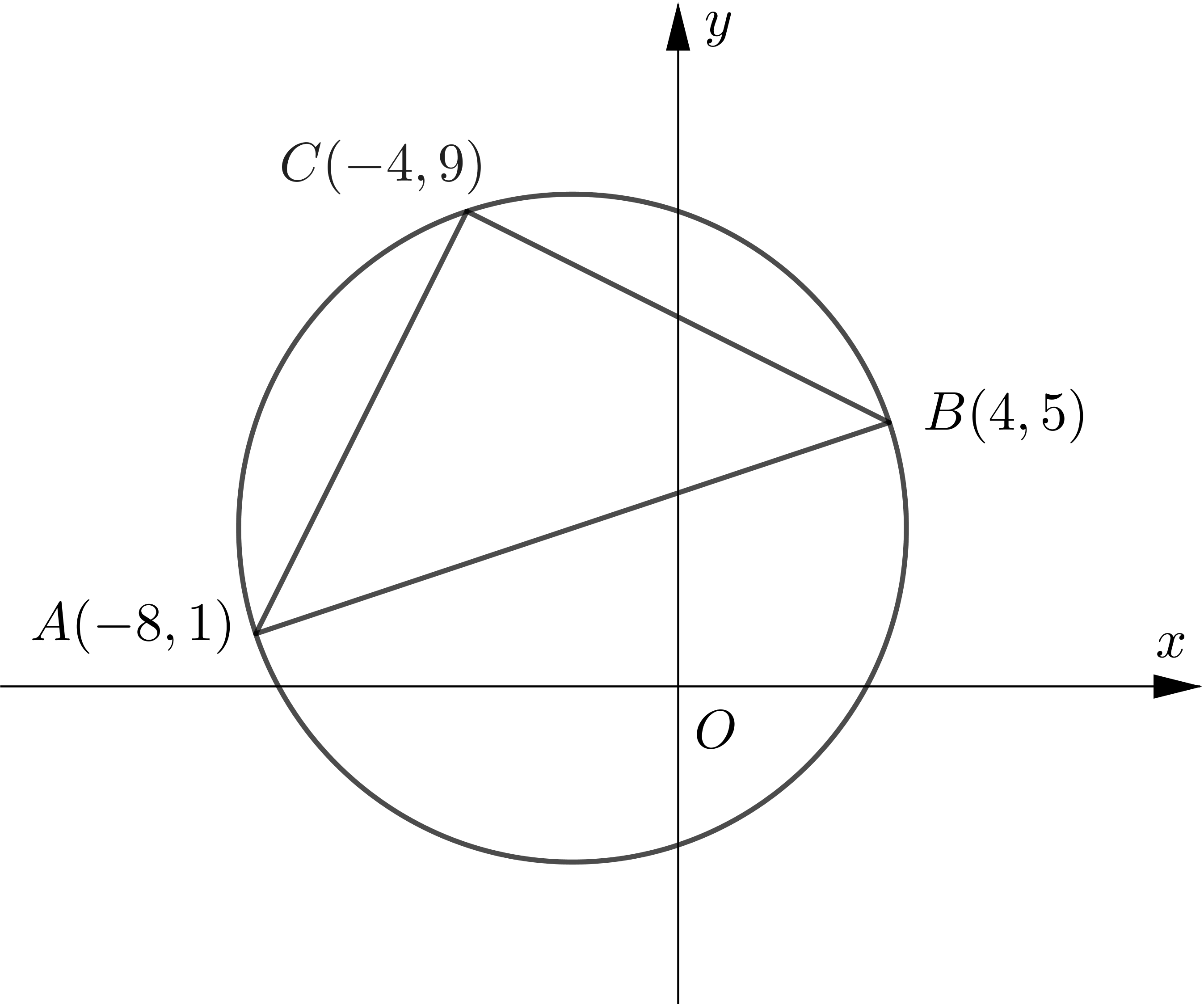The points $A(-8,1), B(4,5)$ and $C(-4,9)$ lie on the circle, as shown in the diagram.
(a) Show that $A B$ is a diameter of the circle.
(b) Find an equation of the circle.

\begin{aligned} &A:\ (-8,1) \\\\ &B:\ (4,5) \\\\ &C:\ (-4,9) \\\\ \end{aligned}
\begin{aligned} \text { gradient of } A C &=\dfrac{9-1}{-4+8} \\\\ &=2\\\\ \text { gradient of } B C &=\dfrac{9-5}{-4-4} \\\\ &=-\dfrac{1}{2}\\\\ \therefore\ \text { gradient of } & A C \times \text { gradient of } B C\\\\ &=2 \times\left(-\dfrac{1}{2}\right)\\\\ &=-1 \\\\ \end{aligned}
$\therefore A C \perp B C\\\\$
$\therefore\ A B \text { is a diameter. }\\\\$
Let $P$ be the midpoint of $A B.\\\\$
$\therefore P$ is the centre of circle.
\begin{aligned} &\\ P &=\left(\dfrac{-8+4}{2}, \dfrac{1+5}{2}\right)=(-2,3) \\\\ \therefore\ P C &=\sqrt{(-2+4)^{2}+(3-9)^{2}} \\\\ &=\sqrt{4+36} \\\\ &=\sqrt{40}\\\\ \end{aligned}
Thus, the equation of the circle is
\begin{aligned} &\\ (x+2)^{2}+(y-3)^{2}&=40 \end{aligned}

2.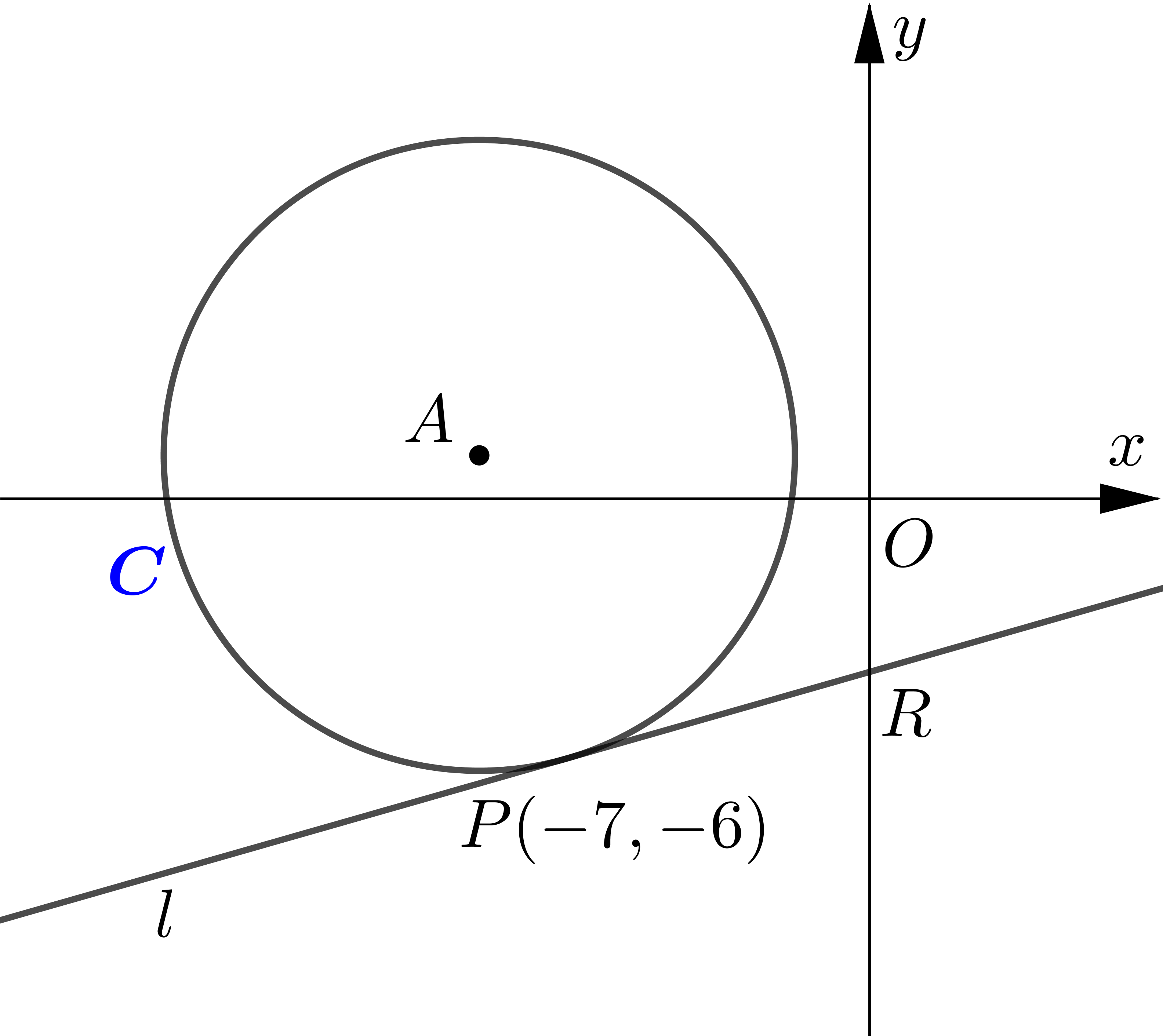The circle $C$ has equation $x^{2}+18 x+y^{2}-2 y+29=0$.
(a) Verify the point $P(-7,-6)$ lies on $C$.
(b) Find an equation for the tangent to $C$ at the point $P$, giving your answer in the form $y=m x+b$.
(c) Find the coordinates of $R$, the point of intersection of the tangent and
(d)Find the area of the triangle $A P R$.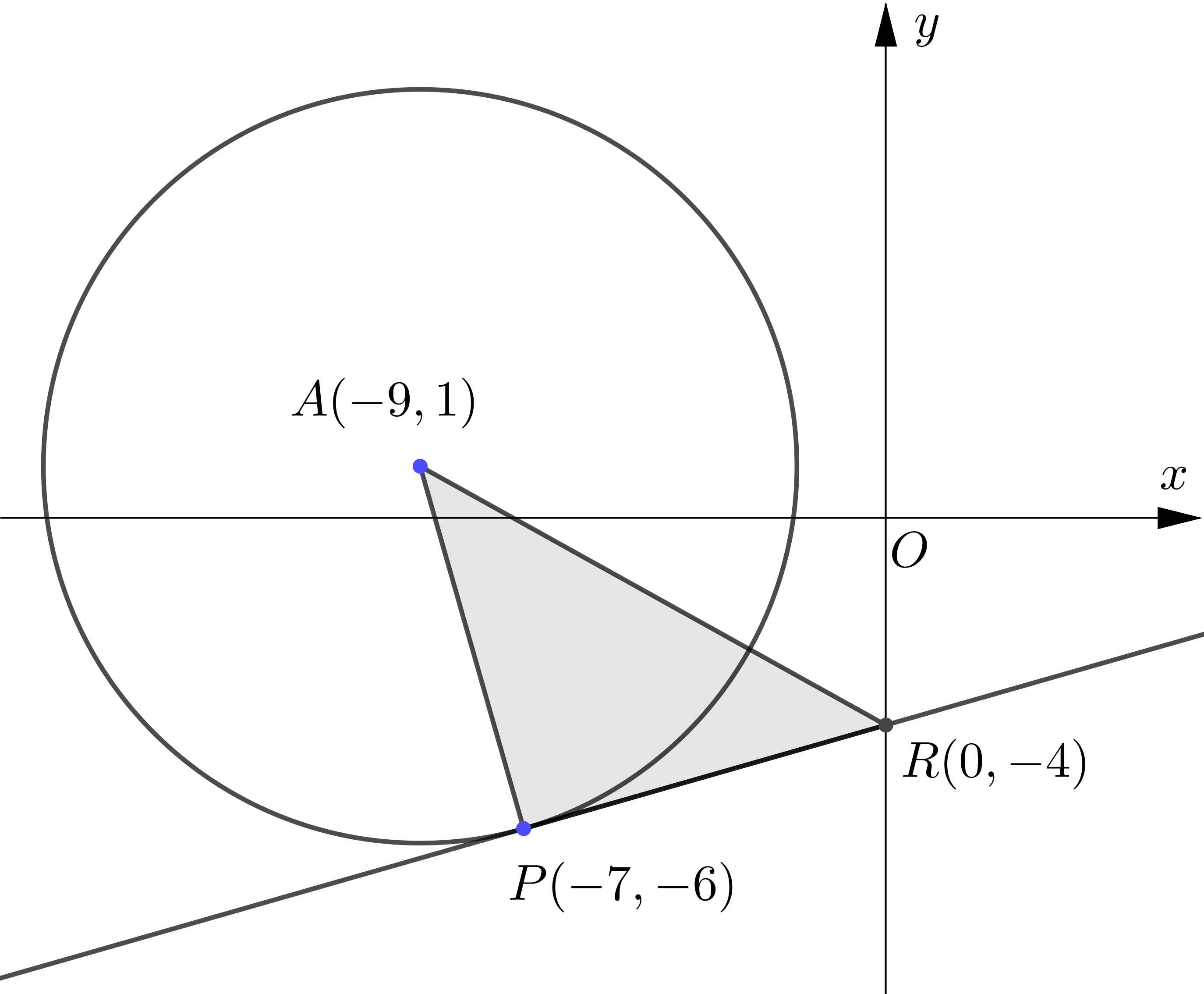$C: x^{2}+18 x+y^{2}-2 y+29=0\\\\$
When $x=-7, y=-6\\\\$
\begin{aligned} &(-7)^{2}+18(-7)+(-6)^{2}-2(-6)+29\\\\ =&\ 49-126+36+12+29\\\\ =&\ 0\\\\ \end{aligned}
$\therefore(-7,-6)$ lies on $C .\\\\$
$C: x^{2}+18 x+y^{2}-2 y+29=0\\\\$
$x^{2}+18 x+81+y^{2}-2 y+1=-29+81+1\\\\$
$(x+9)^{2}+(y-1)^{2}=53\\\\$
$\therefore$ centre $(A):(-9,1)\\\\$
\begin{aligned} \text{gradent of } A P &=\dfrac{1+6}{-9+7} \\\\ &=-\dfrac{7}{2} \\\\ \text{gradient of tangent at } P&=\dfrac{2}{7}\\\\ \end{aligned}
$\therefore$ Equation of tangent at $P$ is
\begin{aligned} &\\ y+6 &=\dfrac{2}{7}(x+7) \\\\ \therefore y&=\dfrac{2}{7} x-4\\\\ \end{aligned}
When the tangent cuts $y$ axis, $x=0\\\\$.
\begin{aligned} \therefore\ y &=\dfrac{2}{7}(0)-4=-4 \\\\ \therefore\ R: &(0,-4) \\\\ A P &=\text { length of radius } \\\\ &=\sqrt{53} \\\\ P R &=\sqrt{(0+7)^{2}+(-4+6)^{2}} \\\\ &=\sqrt{53}\\\\ &\text{area of } \triangle A P R\\\\ &=\dfrac{1}{2} \times A P \times P R \\\\ &=\dfrac{1}{2} \times \sqrt{53} \times \sqrt{53} \\\\ &=\dfrac{53}{2} \end{aligned}

3. The tangent to the circle $(x+4)^{2}+(y-1)^{2}=242$ at $(7,-10)$ meets the $y$-axis at $S$ and the $x$-axis at $T$.
(a) Find the coordinates of $S$ and $T$.
(b) Hence, find the area of $\triangle O S T$, where $O$ is the origin.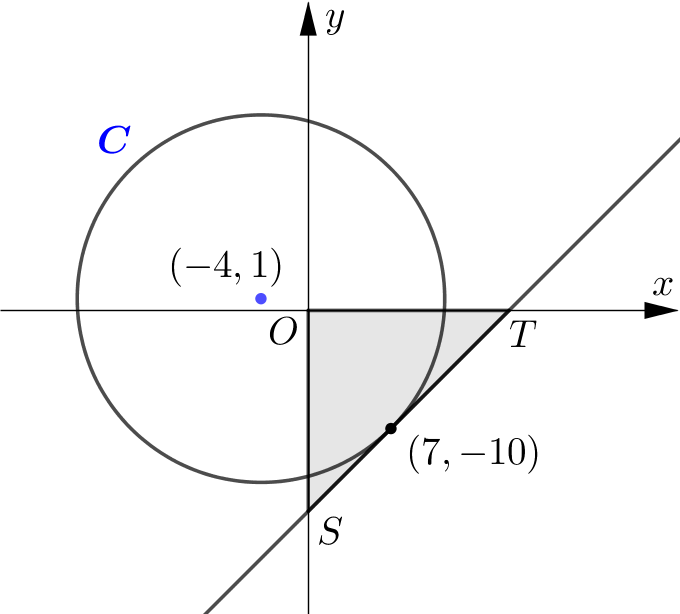Circle: $(x+4)^{2}+(y-1)^{2}=2 y 2\\\\$
Centre: $(-4,1)\\\\$
Tangent point: $(7,-10)\\\\$
let $m_{1}$ be the gradient of the line parsing through $(-4,1)$ and $(7,-10)\\\\$.
$m_{1}=\dfrac{-10-1}{7+4}=-1\\\\$
Let the gradient of toungent to circle at $(7,-10)$ be $m_{2}.\\\\$
$\therefore m_{2}=-\dfrac{1}{m_{1}}=1 .\\\\$
Let the coordinates of the point $S$ and $T$ be $(0, b)$ and $(a, 0)\\\\$.
\begin{aligned} \therefore \dfrac{b-(-10)}{0-7} &=1 \\\\ b &=-17 \\\\ \dfrac{0-(-10)}{a-7} &=1 \\\\ a &=17 \\\\ S &=(0,-17) \\\\ T &=(17,0)\\\\ \text{area of }\triangle OST &=\dfrac{1}{2}|a||b| \\\\ &=\dfrac{1}{2}|17|-17 \mid \\\\ &=\dfrac{289}{2} \end{aligned}

4.The circle $C$ has equation $(x+5)^{2}+(y+3)^{2}=80$. The line $l$ is a tangent to the circle and has gradient $2 .$ Find two possible equations for $l$ giving your answers in the form $y=m x+c$.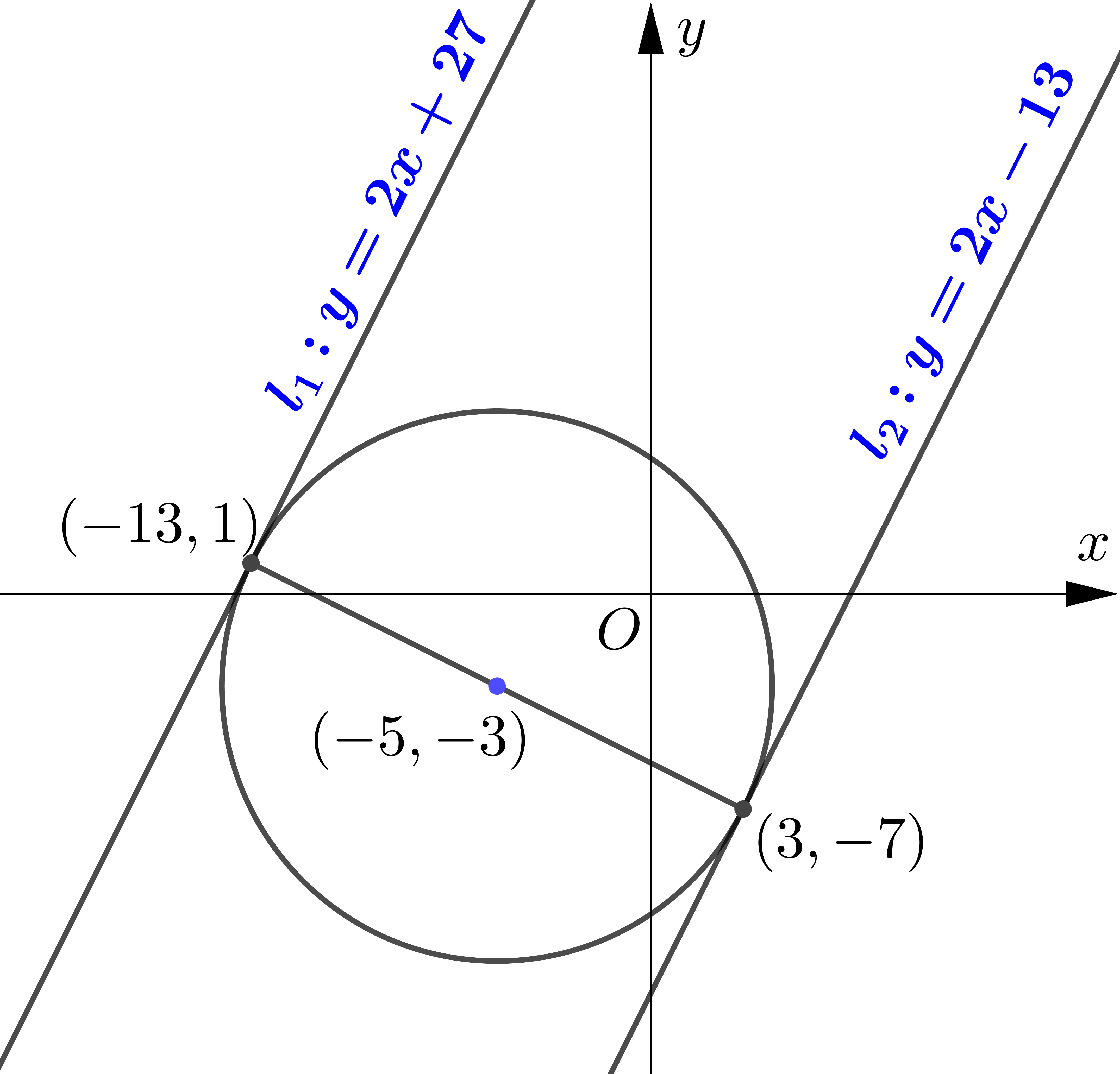$C:(x+5)^{2}+(y+3)^{2}=80\\\\$
Centre: $(-5,-3)\\\\$
radius $=\sqrt{80}\\\\$
Let the gradient of tangent be $m.\\\\$
$\therefore m=2\\\\$
Let the point where the tangent touches the circle be $(a, b).\\\\$
\begin{aligned} \therefore\ \dfrac{b+3}{a+5} &=-\dfrac{1}{2} \\\\ 2 b+6 &=-a-5 \\\\ a &=-2 b-11\\\\ \end{aligned}
$(a, b)$ lies on circle.
\begin{aligned} &\\ \therefore(a+5)^{2}+(b+3)^{2}&=80 \\\\ (-2 b-11+5)^{2}+(b+3)^{2}&=80\\\\ \end{aligned}
Solving equation, $b=-7$ or $b=1\\\\$
\begin{aligned} b=-7 \Rightarrow & a=-2(-7)-11=3 \\\\ b=1 \Rightarrow & a=-2(1)-11=-13\\\\ \end{aligned}
$\therefore$ At $(3,-7)$, the equation of tangent is
\begin{aligned} &\\ y+7 &=2(x-3) \\\\ y &=2 x-13\\\\ \end{aligned}
At $(-13,1)$, the equation of tangent is
\begin{aligned} &\\ y-1&=2(x+13) \\\\ y&=2 x+27 \end{aligned}

5. The line with equation $2 x+y-5=0$ is a tangent to the circle with equation $(x-3)^{2}+(y-p)^{2}=5$.
(a) Find the two possible values of $p$.
(b) Write down the coordinates of the centre of the circle in each case.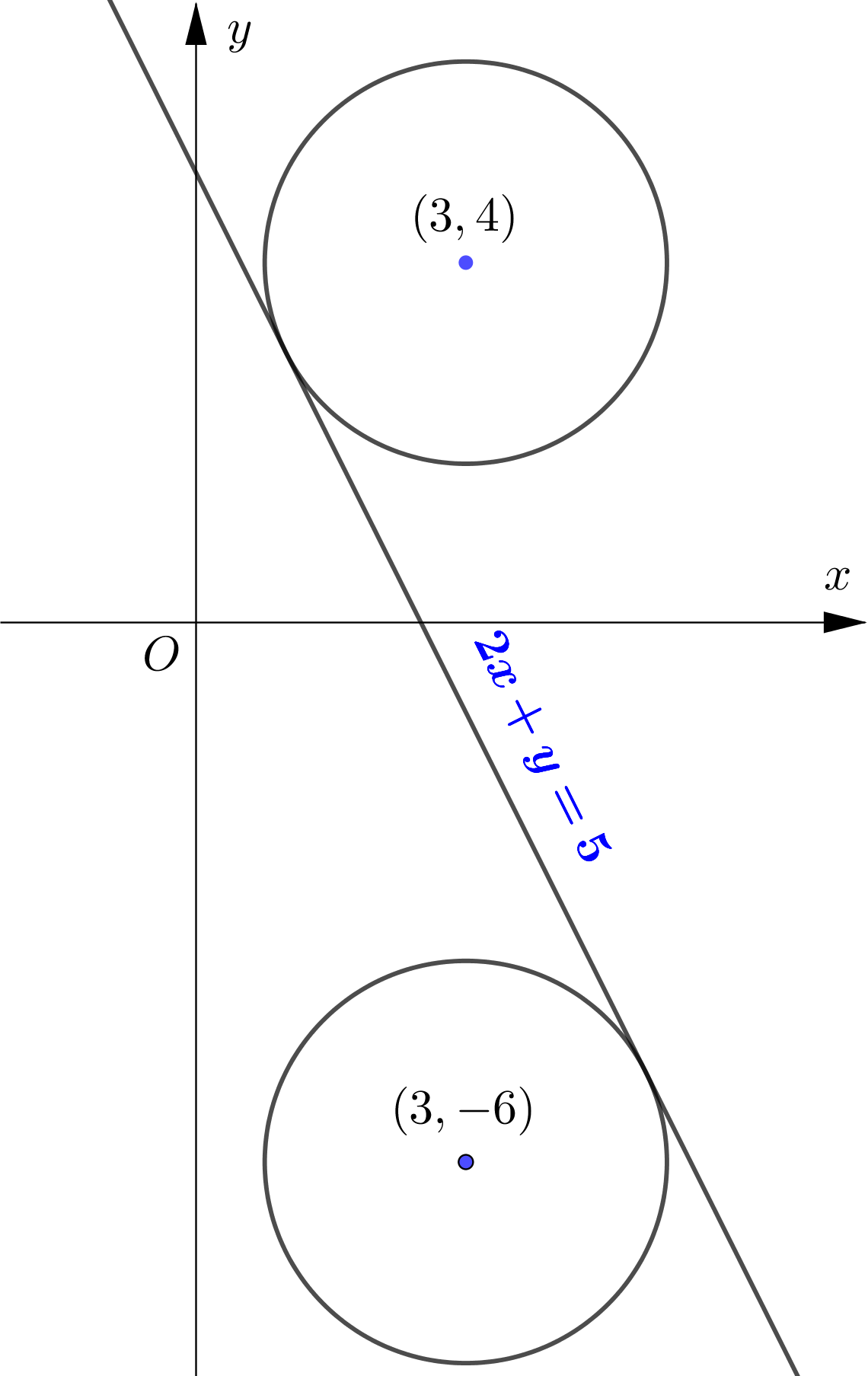### Method 1

Line: $2 x+y-5=0\\\\$
$y=5-2 x\\\\$
$\therefore$ gradient of tangent $\left(m_{1}\right)=-2\\\\$
Circle: $(x-3)^{2}+(y-p)^{2}=5\\\\$
$\therefore$ centre: $(3, p)\\\\$
radius $=\sqrt{5}\\\\$
Let the point of tangency be $(a, 5-2 a).\\\\$
$\therefore$ The gradient of the line passing through $(a, 5-2 a)$ and $(3, p)$ is $\dfrac{1}{2}.\\\\$
\begin{aligned} \therefore \dfrac{5-2 a-p}{a-3} &=\dfrac{1}{2} \\\\ 10-4 a-2 p &=a-3 \\\\ 5 a &=13-2 p \\\\ a &=\dfrac{13-2 p}{5}\\\\ \end{aligned}
$(a, 5-2 a)$ lies on circle.
\begin{aligned} &\\ \therefore(a-3)^{2}+(5-2 a-p)^{2} &=5 \\\\ \left(\dfrac{13-2 p}{5}-3\right)^{2}+\left(5+\dfrac{4 p-26}{5}-p\right)^{2} &=5 \\\\ \left(\dfrac{-2 p-2}{5}\right)^{2}+\left(\dfrac{-p-1}{5}\right)^{2} &=5 \\\\ \dfrac{4}{25}(p+1)^{2}+\dfrac{1}{25}(p+1)^{2} &=5\\\\ \end{aligned}
\begin{aligned} \therefore(p+1)^{2} &=25 \\\\ p+1 &=\pm 5 \\\\ \therefore p &=-6 \text { or } p=4 \\\\ \text { Whan } p &=-6 \text {, centre }=(3,-6). \\\\ \text { When } p &=2 \text {, centre }=(3,4).\\\\ \end{aligned}

### Method 2

Line: $2 x+y-5=0\\\\$
$y=5-2 x\\\\$
Circle: $(x-3)^{2}+(y-p)^{2}=5\\\\$
$\therefore$ Centre: $(3, p).\\\\$
At the point of tangency,
\begin{aligned} &\\ (x-3)^{2}+(5-2 x-p)^{2}&=5\\\\ x^{2}-6 x+9+(5-2 x)^{2}-2 p(5-2 x)+p^{2}&=5 \\\\ x^{2}-6 x+9+25-20 x+4 x^{2}-10 p+4 p x+p^{2}-5&=0 \\\\ 5 x^{2}+(4 p-26) x+\left(p^{2}-10 p+29\right)&=0\\\\ \end{aligned}
Since the tangent touch the circle at only one point, the discriminant $=0.$
\begin{aligned} &\\ (4 p-26)^{2}-4 \times 5\left(p^{2}-10 p+29\right)&=0 \\\\ 16 p^{2}-208 p+676-20 p^{2}+200 p-580&=0 \\\\ -4 p^{2}-8 p+96&=0 \\\\ p^{2}+2 p-24&=0 \\\\ (p+6)(p-4)&=0\\\\ \therefore p =-6 \text { or } p&=4 \\\\ \text { Whan } p =-6 \text {, centre }&=(3,-6). \\\\ \text { When } p =2 \text {, centre }&=(3,4). \end{aligned}

6.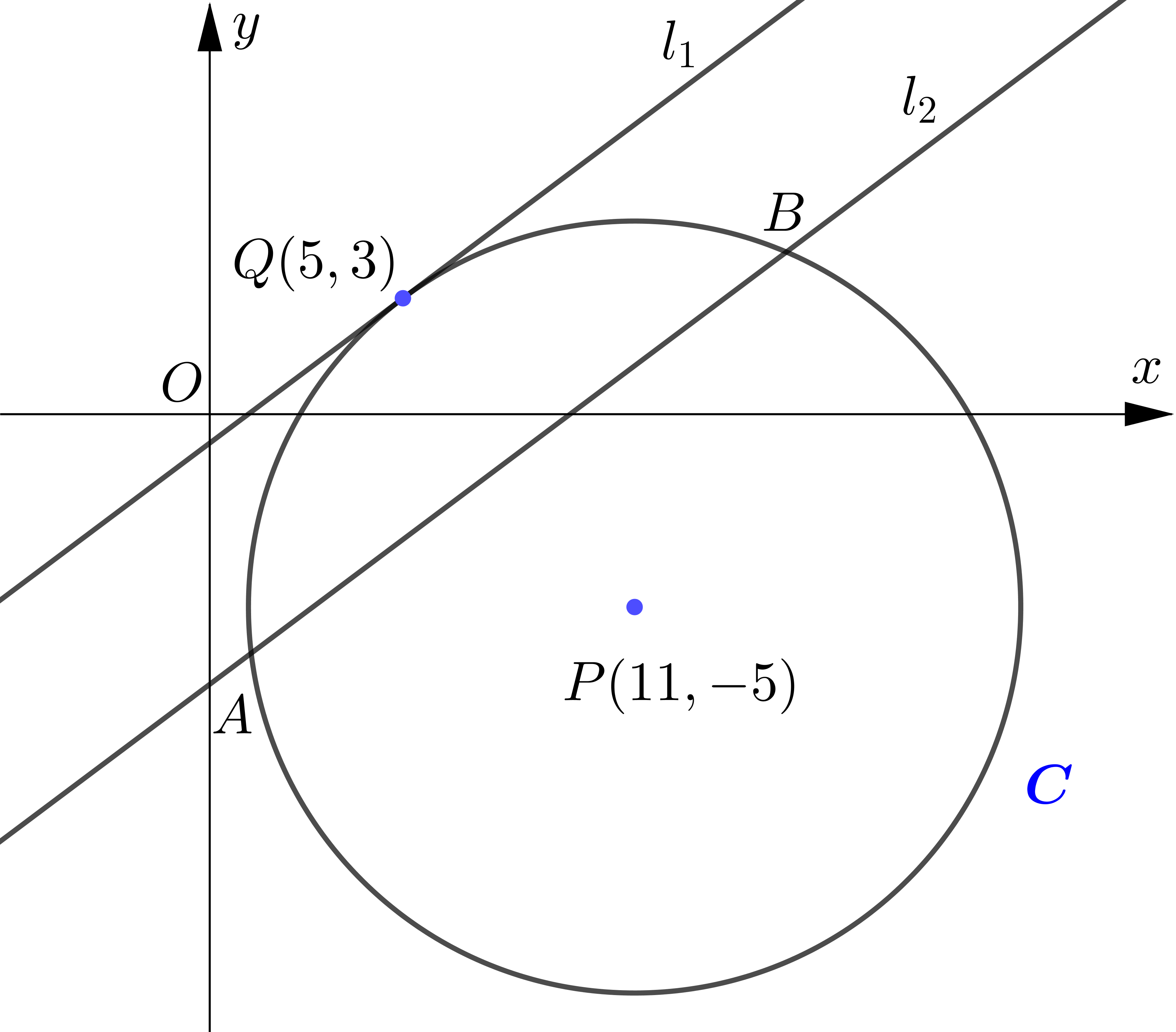The circle $C$ has centre $P(11,-5)$ and passes through the point $Q(5,3)$.
(a) Find an equation for $C$.
The line $l_{1}$ is a tangent to $C$ at the point $Q$.
(b) Find an equation for $l_{1}$.
The line $l_{2}$ is parallel to $l_{1}$ and passes through the midpoint of $P Q .$
(c) Find the equation of $l_2$.
Given that $l_{2}$ intersects $C$ at $A$ and $B$. find the coordinates of points $A$ and $B$,
(d) find the length of the line segment $A B$, leaving your answer in its simplest surd form.

Circle $C$ has centre at $P(11,-5)$ and passes through the point $Q(5,3)\\\\$.
\begin{aligned} \therefore \text { radius } &=\sqrt{(11-5)^{2}+(-5-3)^{2}} \\\\ &=\sqrt{100} \\\\ &=10\\\\ \end{aligned}
$\therefore$ Equation of $C$ is
\begin{aligned} &\\ (x-11)^{2}+(y+5)^{2}&=100\\\\ \end{aligned}
\begin{aligned} \text { Gracient of } P Q &=\dfrac{3+5}{5-11} \\ &=-\dfrac{4}{3}\\\\ \end{aligned}
$\therefore$ Gradient of tangent at $Q=\dfrac{3}{4}\\\\$
Equation of tangent $l_{1}$ at $Q$ is
\begin{aligned} &\\ y-3 &=\dfrac{3}{4}(x-5) \\\\ 4 y-12 &=3 x-15 \\\\ 3 x-4 y &=3\\\\ \end{aligned}
Midpoint of $PQ=\left(\dfrac{11+5}{2}, \dfrac{-5+3}{2}\right)=(8,-1)\\\\$
The equation line $l_{2}$ prallel to $l_1$ and passing through $(8,-1)$ is
\begin{aligned} &\\ y+1&=\dfrac{3}{4}(x-8) \\\\ y&=\dfrac{3 x-28}{4}\\\\ \end{aligned}
When $l_{2}$ intersects $C$.
\begin{aligned} &\\ &(x-11)^{2}+\left(\dfrac{3 x-28}{4}+5\right)^{2}=100 \\\\ &(x-11)^{2}+\left(\dfrac{3 x-8}{4}\right)^{2}=100 \\\\ &x^{2}-22 x+121+\dfrac{9 x^{2}-48 x+64}{16}=100 \\\\ &16 x^{2}-352 x+1936+9 x^{2}-48 x+64=1600 \\\\ &25 x^{2}-400 x+400=0 \\\\ &x^{2}-16 x+16=0\\\\ \end{aligned}
$x^{2}-16 x+64=48\\\\$
$(x-8)^{2}=48\\\\$
$x-8=\pm 4 \sqrt{3}\\\\$
$x=8 \pm 4 \sqrt{3}\\\\$
When $x=8-4 \sqrt{3}\\\\$
$y=\dfrac{1}{4}(24-12 \sqrt{3}-28)\\\\$
$=-1-3 \sqrt{3}\\\\$
\begin{aligned} \text { When } x&=8+4 \sqrt{3}, \\\\ y &=\dfrac{1}{4}(24+12 \sqrt{3}-28) \\\\ &=-1+3 \sqrt{3}\\\\ \end{aligned}
$\therefore$ The coordinates of the points $A$ and $B$ ane $(8-4 \sqrt{3}-1-3 \sqrt{3})$ and $(8+4 \sqrt{3},-1+3 \sqrt{3})$.
\begin{aligned} &\\ \therefore\ A B & =\sqrt{(8 \sqrt{3})^2+6(\sqrt{3})^2} \\\\ & =\sqrt{192+108} \\\\ & =\sqrt{300} \\\\ & =10 \sqrt{3} \end{aligned}

7.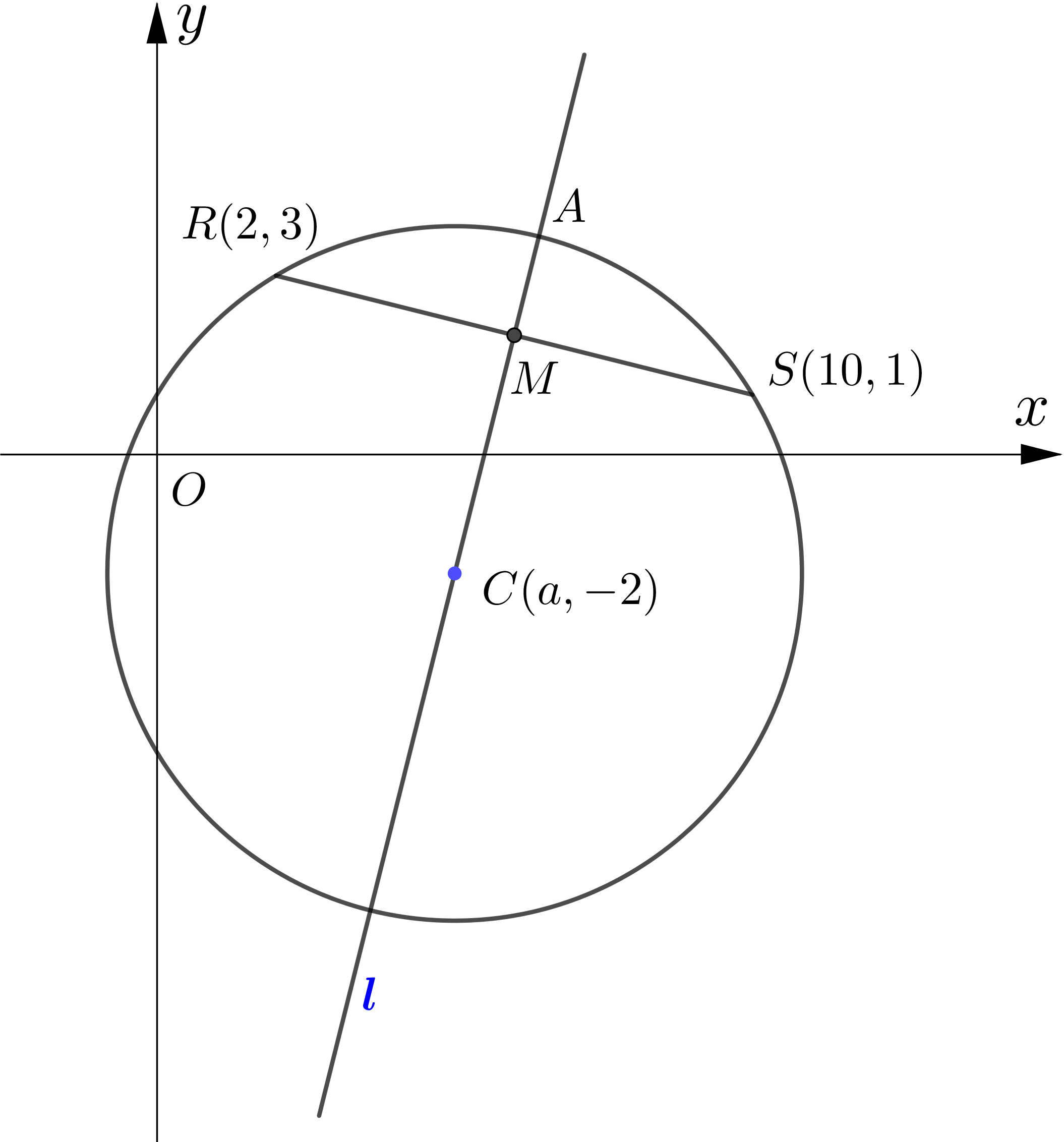The points $R$ and $S$ lie on a circle with centre $C(a,-2)$, as shown in the diagram.The point $R$ has coordinates $(2,3)$ and the point $S$ has coordinates $(10,1) .$ $M$ is the midpoint of the line segment $R S .$ The line $l$ passes through $M$ and $C .$
(a) Find an equation for $l .$
(b) Find the value of $a .$
(c) Find the equation of the circle.
(d) Find the points of intersection, $A$ and $B$, of the line $l$ and the circle.

$M$ is the midpoint of $R S$.
\begin{aligned} &\\ \therefore\ M &=\left(\dfrac{2+10}{2}, \dfrac{3+1}{2}\right) \\\\ &=(6,2)\\\\ \end{aligned}
Gradient of $R S=\dfrac{3-1}{2-10}=-\dfrac{1}{4}$
\begin{aligned} &\\ \therefore \text{Gradient of } l&=4\\\\ \end{aligned}
Equation of $l$ is
\begin{aligned} &\\ y-2 &=4(x-6) \\\\ y &=4 x-22\\\\ \end{aligned}
$l$ passes through $(a,-2)$
\begin{aligned} &\\ \therefore-2 &=4 a-22 \\\\ 4 a &=20 \\\\ a &=5\\\\ \end{aligned}
radius of circle $=R C$
\begin{aligned} &\\ &=\sqrt{(5-2)^{2}+(-2-3)^{2}} \\\\ &=\sqrt{34}\\\\ \end{aligned}
The equation of circle is
\begin{aligned} &\\ (x-5)^{2}+(y+2)^{2}&=34\\\\ \end{aligned}
When $l$ intersects the circle,
\begin{aligned} &\\ &(x-5)^{2}+(4 x-22+2)^{2}=34\\\\ &(x-5)^{2}+(4 x-22+2)^{2} =34 \\\\ &x^{2}-10 x+25+16 x^{2}-160 x+400-34=0 \\\\ &17 x^{2}-170 x+391 =0 \\\\ &x^{2}-10 x+23 =0 \\\\ &x^{2}-10 x+25 =2 \\\\ &(x-5)^{2} =2 \\\\ &x-5 =\pm \sqrt{2} \\\\ &x =5 \pm \sqrt{2}\\\\ \end{aligned}
\begin{aligned} \text { When } x&=5-\sqrt{2}, \\\\ y &=4(5-\sqrt{2})-22 \\\\ &=-4 \sqrt{2}-2\\\\ \text { When } x&=5+\sqrt{2} \\\\ &y=4(5+\sqrt{2})-22 \\\\ &=4 \sqrt{2}-2 \\\\ \therefore\ A&=(5+\sqrt{2}, 4 \sqrt{2}-2) \\\\ B &=(5-\sqrt{2},-4 \sqrt{2}-2) \end{aligned}

8.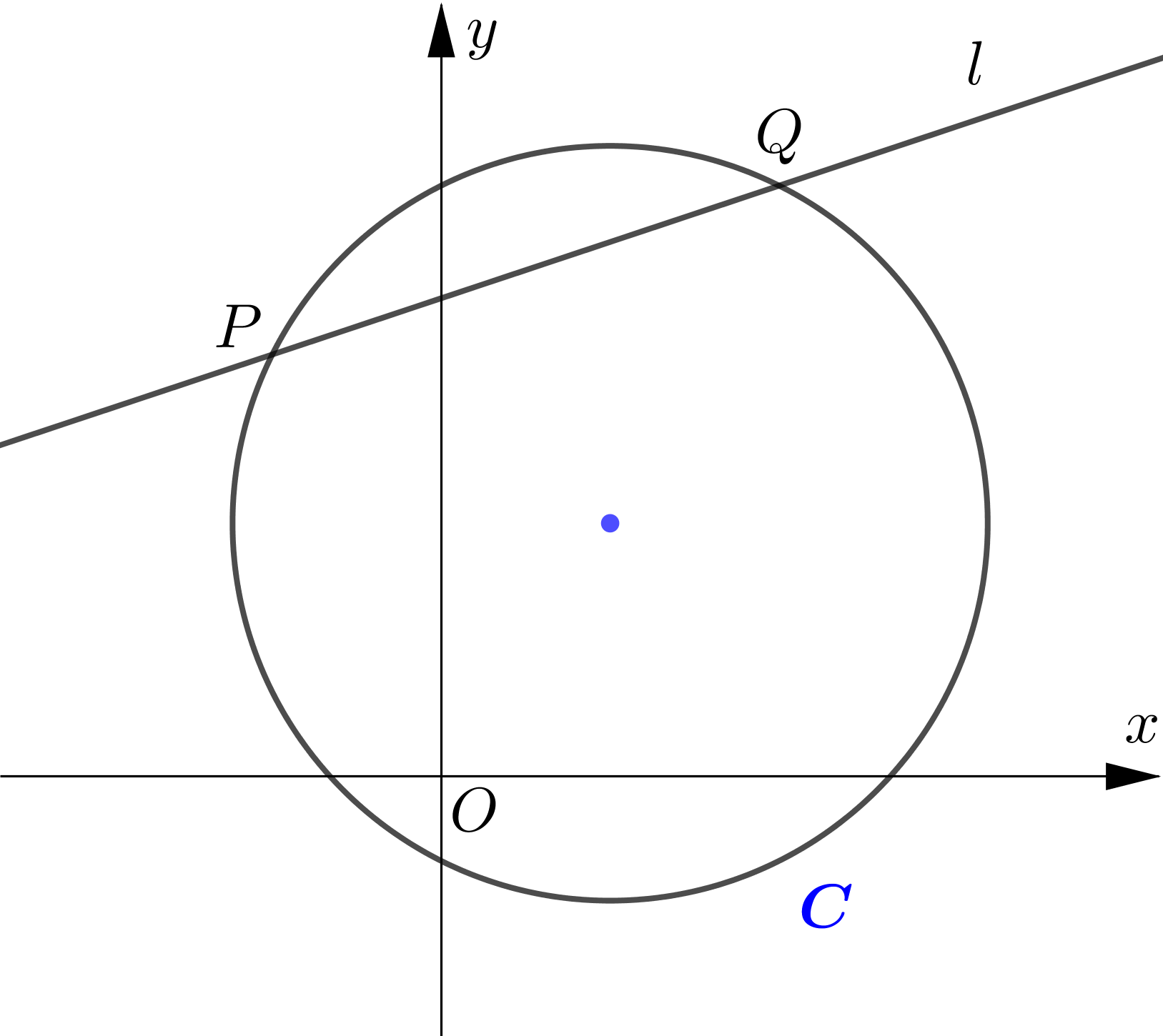The circle $C$ has equation $x^{2}-4 x+y^{2}-6 y=7$. The line $l$ with equation $x-3 y+17=0$ intersects the circle at the points $P$ and $Q$.
(a) Find the coordinates of the point $P$ and the point $Q$.
(b) Find the equation of the tangent at the point $P$ and the point $Q$.
(c) Find the equation of the perpendicular bisector of the chord $P Q$.
(d) Show that the two tangents and the perpendicular bisector intersect at a single point and find the coordinates of the point of intersection.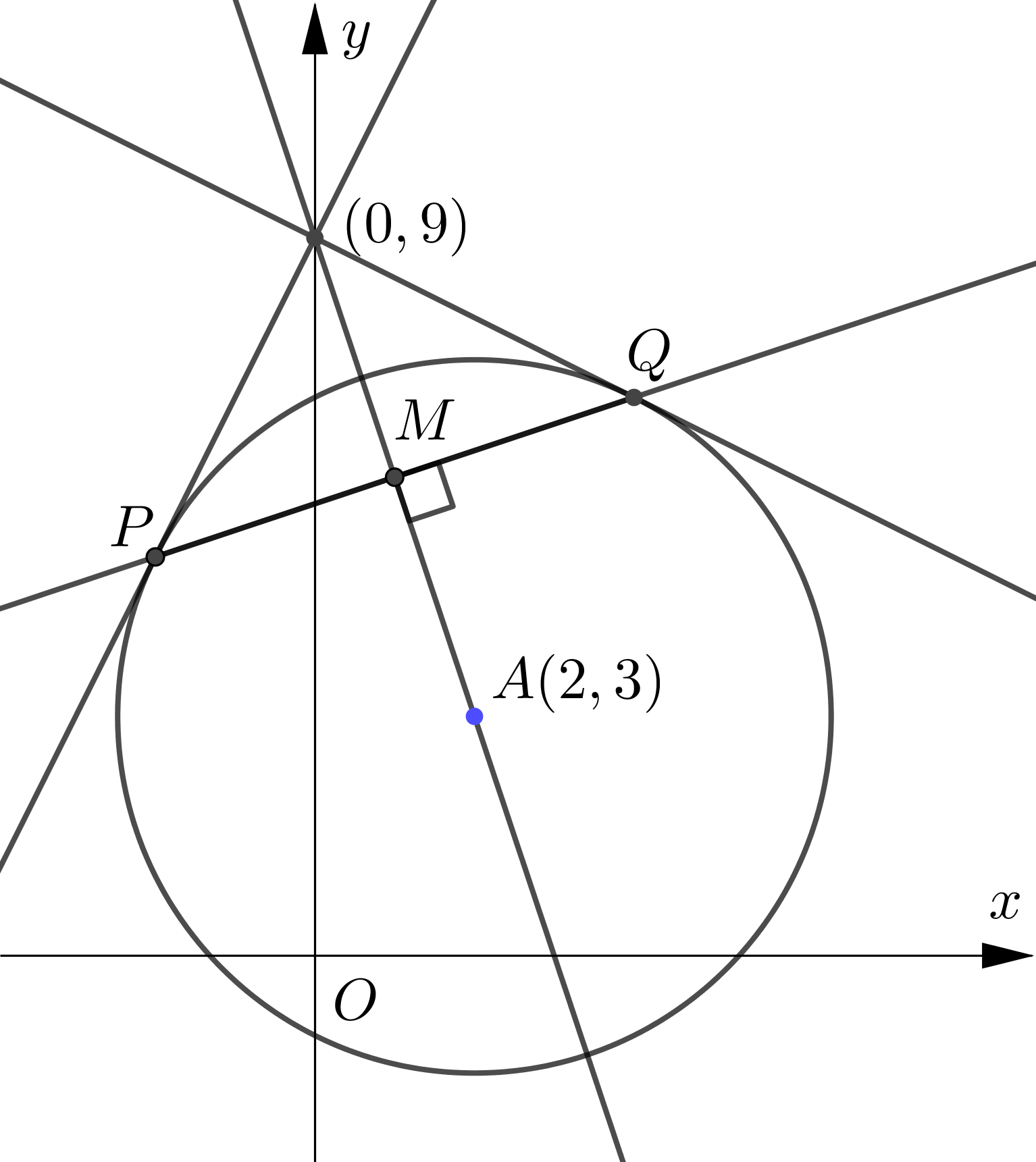\begin{aligned} &C: x^{2}-4 x+y^{2}-6 y=7 \\\\ &x^{2}-4 x+4+y^{2}-6 y+9=4+9+7 \\\\ &(x-2)^{2}+(y-3)^{2}=20 \\\\ &\therefore\ \text { centre: }(2,3) \\\\ &\text { radius }=\sqrt{20}\\\\ \end{aligned}
Let the centre of the circle be $A$.
\begin{aligned} &\\ \text { gradient of } A P&=\dfrac{3-5}{2-(-2)}=-\dfrac{1}{2}\\\\ \end{aligned}
gradient of tangent at $P=2$
\begin{aligned} &\\ \text { gradient of } A Q &=\dfrac{3-7}{2-4}=2\\\\ \end{aligned}
gradient of tangent at $Q=-\dfrac{1}{2}\\\\$
Equation of tangent at $P$ is
\begin{aligned} &\\ y-5 &=2(x+2) \\\\ y &=2 x+9\\\\ \end{aligned}
Equation of tangent at $Q$ is
\begin{aligned} &\\ y-7&=-\dfrac{1}{2}(x-4) \\\\ y&=-\dfrac{1}{2} x+9\\\\ \end{aligned}
Let the midpoint of $PQ$ be $M$.
\begin{aligned} &\\ \therefore\ M &=\left(\dfrac{-2+4}{2}, \dfrac{5+7}{2}\right) \\\\ &=(1,6)\\\\ \end{aligned}
Gradient of $A M=\dfrac{3-6}{2-1}=-3\\\\$
$\therefore$ Equation of the perpendicular bisector of $PQ$ is
\begin{aligned} &\\ y-6&=-3(x-1) \\\\ y&=-3 x+9\\\\ \end{aligned}
At the point of intersection of two tangents,
\begin{aligned} &\\ 2 x+9 &=-\dfrac{1}{2} x+9 \\\\ \therefore x&=0\\\\ \end{aligned}
Substituting $x=0$ in $y=2 x+9\\\\$,
$y=2(0)+9=9\\\\$
The point of intersection of two tangents is $(0,9).\\\\$
Substituting $x=0$ in $y=-3 x+9\\\\$.
$y=-3(0)+9=9\\\\$
$\therefore$ The two tangents and the perpendicular bisector intersect at a single point.
The point of intersection of the two tangents and the perpendicular bisector is $(0,9)$.

9. The circle with centre $C$ has equation $(x-2)^{2}+(y-1)^{2}=10$. The tangents to the circle at points $P$ and $Q$ meet at the point $R$ with coordinates $(6,-1)$.
(a) Show that $C P R Q$ is a square.
(b) Hence find the equations of both tangents.\begin{aligned} \text { Circle: } & (x-2)^{2}+(y-1)^{2}=10 \\\\ \therefore\ \text{center}:\ & (2,1) \\\\ \text { radius }& =\sqrt{10} \\\\ C R &=\sqrt{(2-6)^{2}+(1+1)^{2}} \\\\ &=\sqrt{16+4} \\\\ &=\sqrt{20}\\\\ &=\sqrt{10} \sqrt{2} \\\\ &=\text { radius } \times \sqrt{2}\\\\ \end{aligned}
Since radius $\perp$ tangent, $\triangle C P R$ and $\triangle C Q R$ are $45^{\circ}-45^{\circ}$ right triangle.
\begin{aligned} &\\ \therefore\ & CPRQ \text{ is a square.}\\\\ \end{aligned}
Let the tangent meet the circle at $P(a, b)$.
\begin{aligned} &\\ &(a-2)^{2}+(b-1)^{2}=10 \\\\ &a^{2}-4 a+4+b^{2}-2 b+1=10 \\\\ &a^{2}+b^{2}-4 a-2 b=5 ---(1)\\\\ &\text { Since } C P=P R, \\\\ &(a-6)^{2}+(b+1)^{2}=10 \\\\ &a^{2}-12 a+36+b^{2}+2 b+1=10 \\\\ &a^{2}+b^{2}-12 a+2 b=-27---(2)\\\\ &(1)-(2) \text{ yields}\\\\ &8 a-4 b=32\\\\ &2 a-b=8\\\\ &b=2 a-8\\\\ \end{aligned}
substituting $b=2 a-8$ in eqn: $(1)$,
\begin{aligned} &\\ &a^{2}+(2 a-8)^{2}-4 a-2(2 a-8)=5 \\\\ &a^{2}+4 a^{2}-32 a+64-4 a-4 a+16-5=0\\\\ &5 a^{2}-40 a+75=0 \\\\ &a^{2}-8 a+15=0 \\\\ &a=3 \text { or } a=5 \\\\ &\text { When } a=3, b=-2 \\\\ &\text { When } a=5, b=2\\\\ \end{aligned}
Let the coordinates of the point $P$ and $Q$ be $(3,-2)$ and $(5,2)\\\\\\$.
gradient of $R P=\dfrac{-2+1}{3-6}=\dfrac{1}{3}\\\\$
$\therefore$ gradient of $R Q=-3\quad (\because RP\perp RQ) \\\\$
The equation of tangent $P R$ is
\begin{aligned} &\\ y+1 &=\dfrac{1}{3}(x-6) \\\\ y &=\dfrac{1}{3} x-3\\\\ \end{aligned}
The equation of tangent $R Q$ is
\begin{aligned} &\\ y+1 &=-3(x-6) \\\\ y &=-3 x+17\\\\ \end{aligned}

10.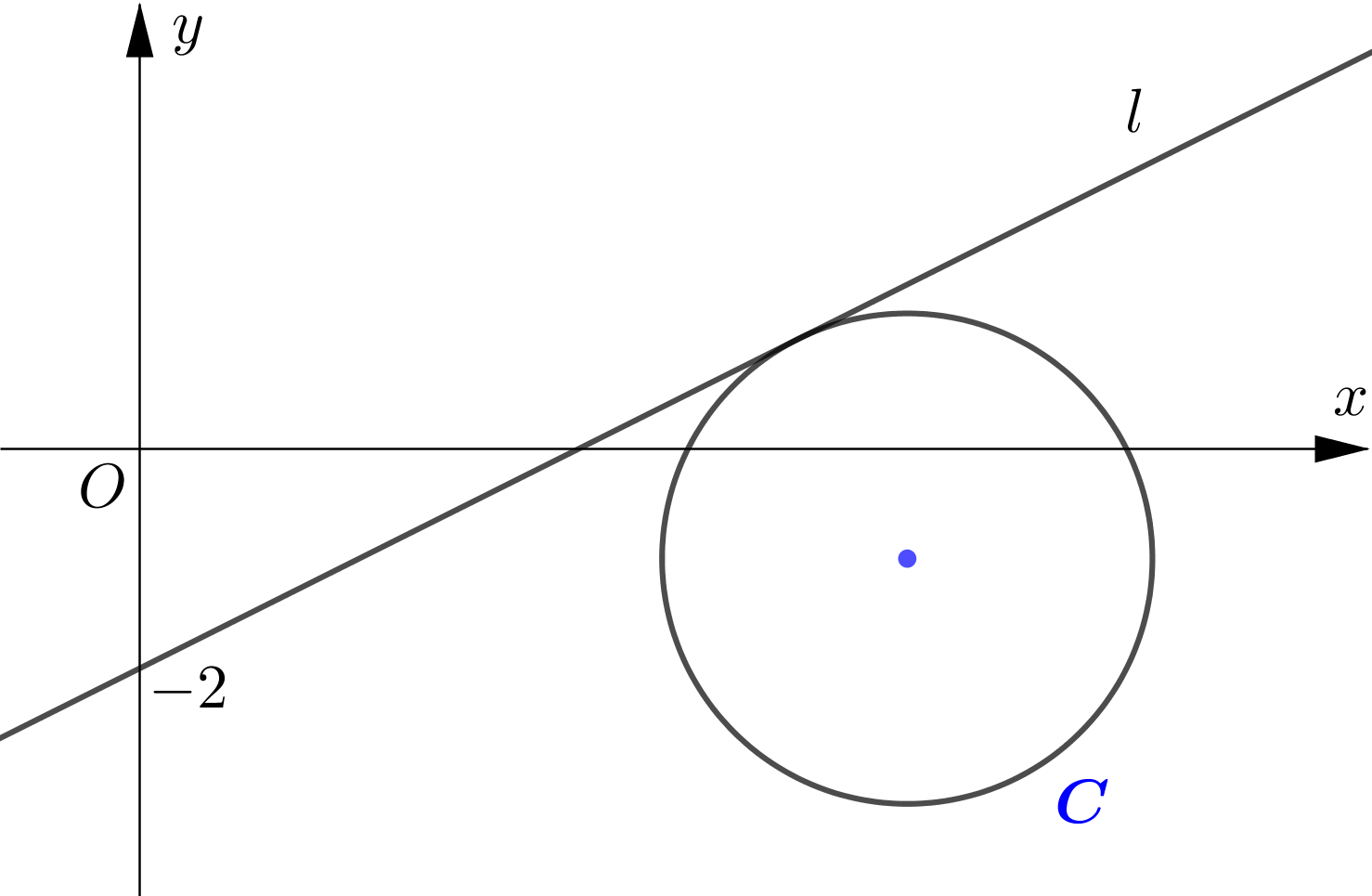The circle $C$ has equation $(x-7)^{2}+(y+1)^{2}=5$. The line $l$ with positive gradient passes through $(0,-2)$ and is a tangent to the circle. Find an equation of $l$, giving your answer in the form $y=m x+c$.

Circle: $(x-7)^{2}+(y+1)^{2}=5\\\\$
Centre: $(7,-1)\\\\$
Let $Q=(0,-2)$ and $l$ touch the circle at $(a, b).\\\\$
$\therefore\ (a-7)^{2}+(b+1)^{2}=5\\\\$
$a^{2}-14 a+49+b^{2}+2 b+1=5\\\\$
$a^{2}+b^{2}-14 a+2 b=-45 --- (1)\\\\$
\begin{aligned} P Q^{2} &=O Q^{2}-O P^{2} \\\\ a^{2}+(b+2)^{2} &=7^{2}+(-1+2)^{2}-5 \\\\ &=45 \\\\ a^{2}+b^{2}+4 b+4 &=45 \\\\ a^{2}+b^{2}+4 b &=41---(2)\\\\ \end{aligned}
Subtracting eqn: (1) from equation(2),
\begin{aligned} &\\ 14 a+2 b &=86 \\\\ 7 a+b &=43 \\\\ b &=43-7 a\\\\ \end{aligned}
Substitue $b=43-7 a$ in equation (2),
\begin{aligned} &\\ a^{2}+(43-7 a)^{2}+4(43-7 a)&=41 \\\\ 50 a^{2}-630 a+1980&=0 \\\\ \therefore a=6 \text { or } a&=\dfrac{33}{5}\\\\ \end{aligned}
When $a=6, b=1\\\\$
When $a=\dfrac{33}{5}, b=-\dfrac{16}{5}\\\\$
Since the gradient of $l=\dfrac{b+2}{a}>0\\\\$,
we have to choose $a=6$and $b=1\\\\$.
$\therefore$ gradient of $l=\dfrac{1+2}{6}=\dfrac{1}{2}\\\\$
The line equation of $l$ is $y+2=\dfrac{1}{2} x \Rightarrow y=\dfrac{1}{2} x-2\\\\$.

စာဖတ်သူ၏ အမြင်ကို လေးစားစွာစောင့်မျှော်လျက်!